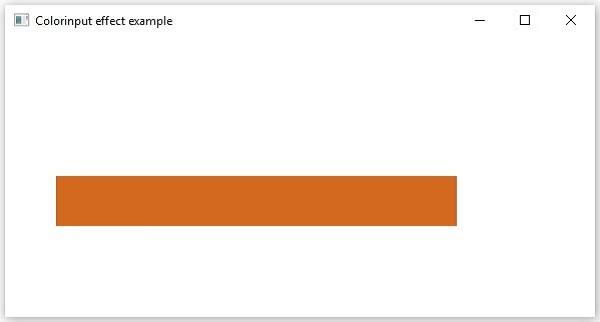# JavaFX Effects - Color Input

Color Input Effect gives the same output as drawing a rectangle and filling it with color. Unlike other effects, if this effect is applied to any node, it displays only a rectangular box (not the node). This effect is mostly used to pass as an input for other effects.

For example, while applying the blend effect, it requires an object of effect type as input. There we can pass this as an input.

The class named ColorInput of the package javafx.scene.effect represents the color input effect. This class contains four properties namely −

• x − This property is of double type; it represents the x coordinate of the position of the color input.

• y − This property is of double type; it represents the y coordinate of the position of the color input.

• height − This property is of double type; it represents the height of the region that is to be filled with color.

• width − This property is of double type; it represents the width of the region that is to be filled with color.

• paint − This property is of Paint type; it represents the color with which the input region is to be filled.

## Example

Following is an example demonstrating the color input effect. In here, we are creating a color input of the dimensions 50, 400 (height, width) at the position 50, 140, and filling it with the color CHOCOLATE.

We are creating rectangle and applying this effect to it. Save this code in a file with the name ColorInputEffectExample.java.

```import javafx.application.Application;
import javafx.scene.Group;
import javafx.scene.Scene;
import javafx.scene.effect.ColorInput;
import javafx.scene.paint.Color;
import javafx.scene.shape.Rectangle;
import javafx.stage.Stage;

public class ColorInputEffectExample extends Application {
@Override
public void start(Stage stage) {
//creating a rectangle
Rectangle rectangle = new Rectangle();

//Instantiating the Colorinput class
ColorInput colorInput = new ColorInput();

//Setting the coordinates of the color input
colorInput.setX(50);
colorInput.setY(140);

//Setting the height of the region of the collor input
colorInput.setHeight(50);

//Setting the width of the region of the color input
colorInput.setWidth(400);

//Setting the color the color input
colorInput.setPaint(Color.CHOCOLATE);

//Applying coloradjust effect to the Rectangle
rectangle.setEffect(colorInput);

//Creating a Group object
Group root = new Group(rectangle);

//Creating a scene object
Scene scene = new Scene(root, 600, 300);

//Setting title to the Stage
stage.setTitle("Sample Application");

stage.setScene(scene);

//Displaying the contents of the stage
stage.show();
}
public static void main(String args[]){
launch(args);
}
}
```

Compile and execute the saved java file from the command prompt using the following commands.

```javac ColorInputEffectExample.java
java ColorInputEffectExample
```

On executing, the above program generates a JavaFX window as shown below.javafx_effects.htm